##CS 662 Theory of Parallel Algorithms Batcher's Sort

[To Lecture Notes Index]
San Diego State University -- This page last updated February 29, 1996, 1996## Parallel Sorting

### Batcher's Odd-Even Sorting Circuit

Let A and B be sorted lists of length n, n is even

Let OM be the sorted list of length n formed by sorting the elements in the odd positions (1, 3, 5, ...) of A and B

Let EM be the sorted list of length n formed by sorting the elements in the even positions (2, 4, 6, ...) of A and B

Let R be the sorted list of size 2n formed by merging A and B.

Let AJ, BJ, OMJ, EMJ, RJ be the J'th element of the corresponding lists A, B, OM, EM, R.

Example
```A 	1	2	3	15	20	21
B	6	7	8	9	10	30

OM	1	3	6	8	10	20
EM	2	7	9	15	21	30
```
```R 	1	2	3	6	7	8	9	10	15	20	21	30
```

Lemma.
R1 = OM1, R2n = EMn
R2k = min(OMk+1, EMk)
R2k+1 = max(OMk+1, EMk)
Comparator Circuit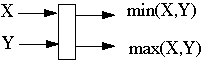N*N Odd-Even Merging CircuitRecursive Definition (N = 4)1*1 Odd-Even Merging Circuit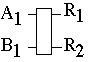2*2 Odd-Even Merging Circuit4*4 Odd-Even Merging Circuit8*8 Odd-Even Merging Circuit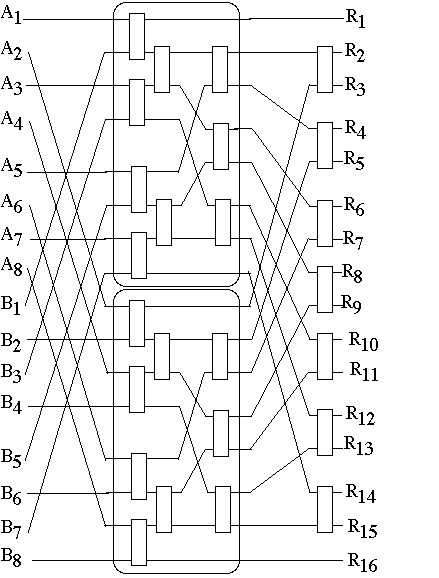#### Complexity Of Odd-Even Merging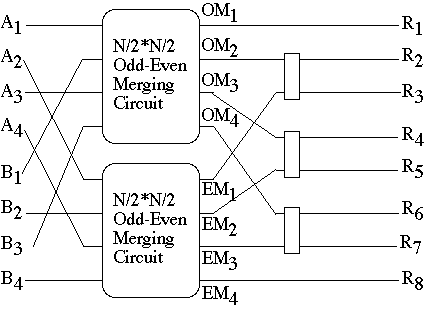Let T(2N) = time required to merge two sorted lists of size N each

Then T(2) = 1 and T(2N) = T(N) + 1

So T(2N) = 1 + lg(N)

Let CC(2N) = the number of comparator circuits needed to merge two sorted lists of size N each

Then CC(2) = 1 and CC(2N) = 2*CC(N) + (N-1)

So CC(2N) = 1 + N*lg(N)

### Sorting By MergingSorting N = 2K items requires K = lg(N) merge passes
N = 8
Pass 1 needs 4 (1*1) merge circuits
time 1
Pass 2 needs 2 (2*2) merge circuits
time 1 + lg(2) = 2
Pass 3 needs 1 (4*4) merge circuits
time 1 + lg(4) = 3

Pass L needs N/2L (2L-1*2L-1) merge circuits time 1 + lg(2L-1) = L

T(N) = time required to sort list of size N = 2K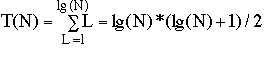Number of Comparator Circuits in Batcher's Odd-Even Sort

Sorting N = 2K items requires K = lg(N) merge passes

Pass L needs N/2L (2L-1*2L-1) merge circuits

(2L-1*2L-1) merge circuit uses 1 + 2L-1*lg(2L-1) = 1 + (L-1)*2L-1 comparator circuits

CC(N) = the number of comparator circuits needed in Batcher's Odd-Even Sort to sort a list of N = 2K items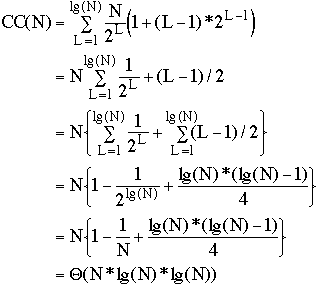Number of Processors in PRAM needed for
Batcher's Odd-Even Sort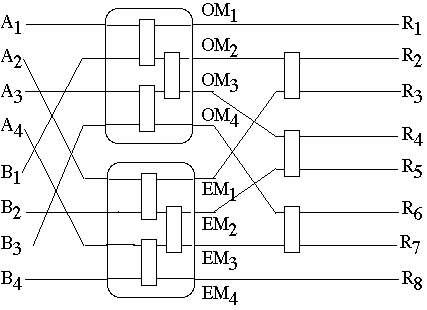P(N) = N/2

T(N) = lg(N)*(lg(N) + 1)/2

C(N) = N*lg(N)*(lg(N) + 1)/4

SN/2(N) = Ts(N)/Tp(N) = 2N/(lg(N) + 1)
Batcher's Odd-Even Sort on PRAM
Batcher's Merge-Exchange Sort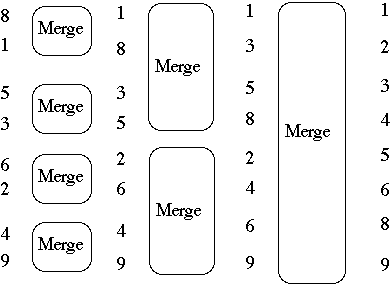8 merge 6 regroup 6 merge 4 merge 1
1 1 1 1 2
5 4 4 5 3
3 3 3 2 4
6 8 8 6 5
2 2 2 3 6
4 5 5 8 8
9 9 9 9 9
Why?
Straighten out some wiresMakes the indexing easier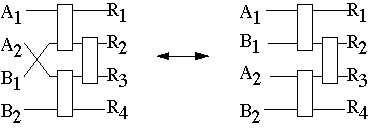Batcher's Merge-Exchange Sort

Input: N and array A[1:N] of items to sort

setfor P = 2T-1, 2T-2, 2T-3, ..., 1 do
R = 0, D = P
for Q = 2T-1, 2T-2, 2T-3, ..., P do
for (K = 1 to N - D ) and ((K-1)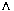P) = R do in parallel
if A[K] > A[K + D] then
swap(A[K], A[K + D ])
end if
end for
D = Q - P
R = P
end for
end for

(K + 1)P means logical and of K and P

If number of processors is less than N than the swap becomes a merge